### Total 125 Videos found in Category "Math Skills"

Page: 1 2 3
Title
 1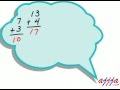1 Digit Addition 1 2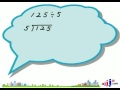1 Digit Division 1 3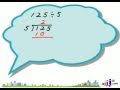1 Digit Division 1_1 4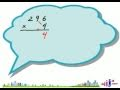1 Digit Multiplication 1 51 Digit Subtraction 1 6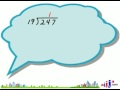2 Digit Division 1 7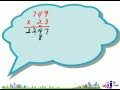2 Digit Multiplication 1 8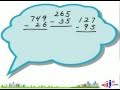2 Digit Subtraction 1 9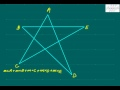Angle of a Star 1 10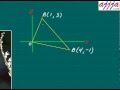Area of Triangle on Graph using Determinant 1 11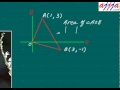Area of Triangle on Graph using Determinant 2 12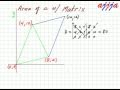Area of Triangle on Graph with Matrix 13Area with 2 Lines in Linear Graph 1 14Average Speed 1 15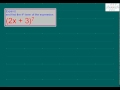Binomial Theorem 1 16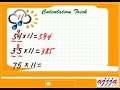Calculation Trick - 11 Times 17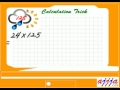Calculation Trick - 125 Times 18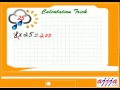Calculation Trick - 25 Times 19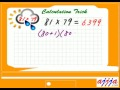Calculation Trick - 81x79 20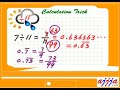Calculation Trick - Divide by 11 21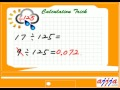Calculation Trick - Divide by 125 22Calculation Trick - Divide by 25 23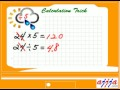Calculation Trick - Divide by 5 24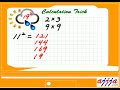Calculation Trick - Just Remember 1 25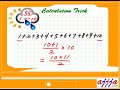Calculation Trick - Sum of Sequence 1 26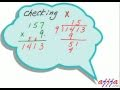Checking - Multiplication Division 1 27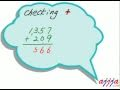Checking Addition Subtraction 1 28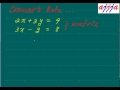Cramers Rule_1 29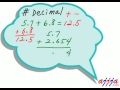Decimal - Addition Subtraction 1 30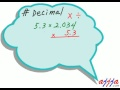Decimal - Multiplication Division 1 31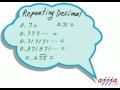Decimal - Repeating 1 32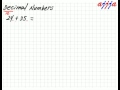Decimal Number Addition Subtraction 1 33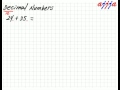Decimal Number Addition Subtraction 1 34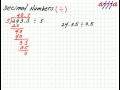Decimal Number Division 1 35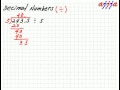Decimal Number Division 1 36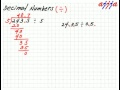Decimal Number Division 10 37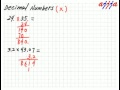Decimal Number Multiplication 1 38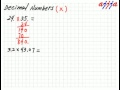Decimal Number Multiplication 1 39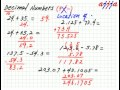Decimal Number Multiplication 10 40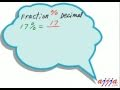Decimal Percent Fraction 1 41Exponential Function 1 42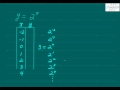Exponential Graph 1 43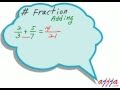Fraction - Addition Subtraction 1 44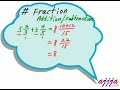Fraction - Addition Subtraction 2 45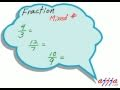Fraction - Mixed Number 1 46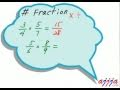Fraction - Multiplication Division 1 47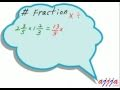Fraction - Multiplication Division 2 48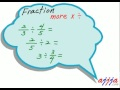Fraction - Multiplication Division 3 49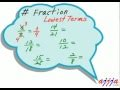Fraction - Reduction 1 50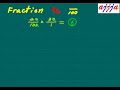Fraction Percent 01

Say and share some thing about these videos...